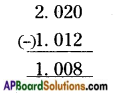AP State Syllabus AP Board 6th Class Maths Solutions Chapter 5 Fractions and Decimals Ex 5.5 Textbook Questions and Answers.

## AP State Syllabus 6th Class Maths Solutions 5th Lesson Fractions and Decimals Ex 5.5

Question 1.
i) 5.702, 5.2, 6.04 and 2.30
ii) 40.004; 44.444; 40.404 and 4.444
i) 5.702, 5.2, 6.04 and 2.30
Given decimals fractions are 5.702; 5.2; 6.04 and 2.30
Convert the given decimals into like decimals.
5.702; 5.200; 6.040; 2.300
Then write the decimals in column with the decimal points directly below each other.
So, that tenth’s come under tenths, hundredths come under hundredths and so on, then add.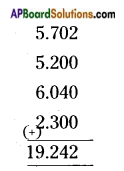ii) 40.004; 44.444; 40.404 and 4.444
Given decimals are 40.004; 44.444; 40.404 and 4.444
They are like decimal fractions.
So, write the decimals in column with the decimal points directly below each other.
So, that tenths come under tenths, hundredths come under hundredths and so on, then add.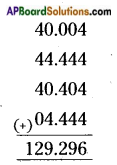Question 2.
Do the following:
i) 426.326 – 284.482
ii) 5 – 3.009
iii) 2.107 – 0.31
i) 426.326 – 284.482
Given decimals are 426.326 and 284.482.
They are like fractions.
So, write the decimals in column with the decimal points directly below each other.
So, that tenths come under tenths, hundredths come under hundredths and so on, then subtract.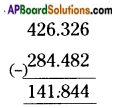ii) 5 – 3.009
Given decimals are 5 and 3.009 Make them as like fractions.
5.000 – 3.009
So, write the decimals in column with the decimal points directly below each other.
So, that tenths come under tenths, hundredths come under hundredths and so on. Then subtract.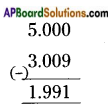iii) 2.107 – 0.31
Given decimals are 2.107 and 0.31, make them as like fractions.
2.107 – 0.310
So, write the decimals in column with the decimal points directly below each other.
So, that tenths come under tenths, hundredths come under hundredths and so on. Then subtract.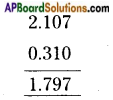Question 3.
Akshara bought 3 m 40 cm cloth for her shirt and 1 m 10 cm cloth for skirt. Find the total cloth bought by her.
Length of cloth bought for shirt = 3.40
Length of cloth bought for skirt = 1.10
Total length of cloth bought by Akshara = 3.40 + 1.10 = 4.50 cm

Question 4.
Write in decimals using the units written in brackets.
i) 90 rupees 75 paisa (Rs.)
ii) 49 m 20 cm (m)
iii) 12 kg 450 g (kg)
iv) 50 l 500 ml (l)
i) 90 rupees 75 paisa = Rs. 90.75
ii) 49 m 20 cm = 49.20 m
iii) 12 kg 450 g = 12.450 kg
iv) 50 l 500 ml = 50.500 lQuestion 5.
i) 58 kg 100 g; 60 kg 350 g
ii) 80 m 15 cm; 72 m 30 cm
i) 58 kg 100 g; 60 kg 350 g
Given values are 58 kg 100 g and 60 kg 350 g
First convert the given values into like decimal fractions.
58.100 kg and 60.350 kg
Then, write the decimals in column with the decimal points directly below each other.
So, that tenths come under tenths, hundredths come under hundredths and so on.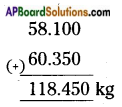ii) 80 m 15 cm; 72 m 30 cm
Given values are 80 m 15 cm and 72 m 30 cm.
First convert the given values into decimal fractions.
80.15 m and 72.30 m
Then, write the decimals in column with the decimal points directly below each other.
So, that tenths come under tenths, hundredths come under hundredths and so on. Then add: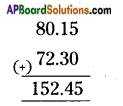Question 6.
Convert into decimals and subtract.
i) 14 kg 720 g from 16 kg 744 g
ii) 1 l 12 ml from 2 l 20 ml
i) 14 kg 720 g from 16 kg 744 g
Given values are 14 kg 720 g and 16 kg 744 g.
First convert the given values into decimal fractions.
14.720 kg and 16.744 kg
Then, write the decimals in column with the decimal points directly below each other. So, that tenths come under tenths, hundredths come under hundredths and so on.
Then subtract: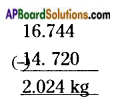ii) 1 l 12 ml from 2 l 20 ml
Given values are 1 l 12 ml and 2 l 20 ml
First convert the given values into decimal fractions.
1.012 l and 2.020 l
Then write the decimals in column with the decimal points directly below each other. So, that tenths come under tenths, hundredths come under hundredths and so on. Then subtract: